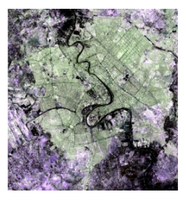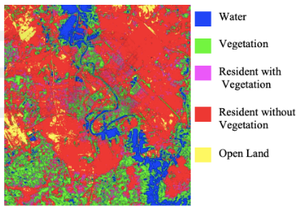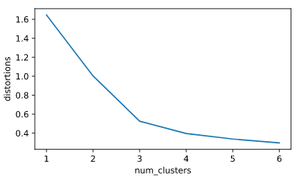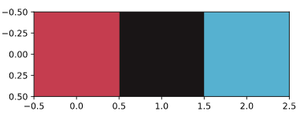# Extract dominant colors of an image using Python

• Difficulty Level : Expert
• Last Updated : 17 May, 2022

Let us see how to extract the dominant colors of an image using Python. Clustering is used in much real-world application, one such real-world example of clustering is extracting dominant colors from an image.

Any image consists of pixels, each pixel represents a dot in an image. A pixel contains three values and each value ranges between 0 to 255, representing the amount of red, green and blue components. The combination of these forms an actual color of the pixel. To find the dominant colors, the concept of the k-means clustering is used. One important use of k-means clustering is to segment satellite images to identify surface features.

Below shown satellite image contains the terrain of a river valley.The terrain of the river valley

Various colors typically belong to different features, k-means clustering can be used to cluster them into groups which can then be identified into various surfaces like water, vegetation etc as shown below.Clustered groups (water, open land, …)

## Tools to find dominant colors

• matplotlib.image.imread – It converts JPEG image into a matrix which contains RGB values of each pixel.
• matplotlib.pyplot.imshow – This method would display colors of the cluster centers after k-means clustering performed on RGB values.

Lets now dive into an example, performing k-means clustering on the following image:Example image

As it can be seen that there are three dominant colors in this image, a shade of blue, a shade of red and black.

Step 1 : The first step in the process is to convert the image to pixels using imread method of image class.

## Python3

 `# Import image class of matplotlib``import` `matplotlib.image as img`` ` `# Read batman image and print dimensions``batman_image ``=` `img.imread(``'batman.png'``)``print``(batman_image.shape)`

Output :

`(187, 295, 4)`

The output is M*N*3 matrix where M and N are the dimensions of the image.

Step 2 : In this analysis, we are going to collectively look at all pixels regardless of there positions. So in this step, all the RGB values are extracted and stored in their corresponding lists. Once the lists are created, they are stored into the Pandas DataFrame, and then scale the DataFrame to get standardized values.

## Python3

 `# Importing the modules``import` `pandas as pd``from` `scipy.cluster.vq ``import` `whiten`` ` `# Store RGB values of all pixels in lists r, g and b``r ``=` `[]``g ``=` `[]``b ``=` `[]``for` `row ``in` `batman_image:``    ``for` `temp_r, temp_g, temp_b, temp ``in` `row:``        ``r.append(temp_r)``        ``g.append(temp_g)``        ``b.append(temp_b)`` ` `# only printing the size of these lists``# as the content is too big``print``(``len``(r))``print``(``len``(g))``print``(``len``(b))`` ` `# Saving as DataFrame``batman_df ``=` `pd.DataFrame({``'red'` `: r,``                          ``'green'` `: g,``                          ``'blue'` `: b})`` ` `# Scaling the values``batman_df[``'scaled_color_red'``] ``=` `whiten(batman_df[``'red'``])``batman_df[``'scaled_color_blue'``] ``=` `whiten(batman_df[``'blue'``])``batman_df[``'scaled_color_green'``] ``=` `whiten(batman_df[``'green'``])`

Output :

```55165
55165
55165```

Step 3 : Now, to find the number of clusters in k-means using the elbow plot approach . This is not an absolute method to find the number of clusters but helps in giving an indication about the clusters.

Elbow plot: a line plot between cluster centers and distortion (the sum of the squared differences between the observations and the corresponding centroid).

Below is the code to generate the elbow plot:

## Python3

 `# Preparing data to construct elbow plot.``distortions ``=` `[]``num_clusters ``=` `range``(``1``, ``7``)  ``#range of cluster sizes`` ` `# Create a list of distortions from the kmeans function``for` `i ``in` `num_clusters:``    ``cluster_centers, distortion ``=` `kmeans(batman_df[[``'scaled_color_red'``, ``                                                    ``'scaled_color_blue'``, ``                                                    ``'scaled_color_green'``]], i)``    ``distortions.append(distortion)``     ` `# Create a data frame with two lists, num_clusters and distortions``elbow_plot ``=` `pd.DataFrame({``'num_clusters'` `: num_clusters,``                           ``'distortions'` `: distortions})`` ` `# Create a line plot of num_clusters and distortions``sns.lineplot(x ``=` `'num_clusters'``, y ``=` `'distortions'``, data ``=` `elbow_plot)``plt.xticks(num_clusters)``plt.show()`

Elbow plot is plotted as shown below :

Output :Elbow plot

It can be seen that a proper elbow is formed at 3 on the x-axis, which means the number of clusters is equal to 3 (there are three dominant colors in the given image).

Step 4 : The cluster centers obtained are standardized RGB values.

`Standardized value = Actual value / Standard Deviation`

Dominant colors are displayed using imshow() method, which takes RGB values scaled to the range of 0 to 1. To do so, you need to multiply the standardized values of the cluster centers with there corresponding standard deviations. Since the actual RGB values take the maximum range of 255, the multiplied result is divided by 255 to get scaled values in the range 0-1.

## Python3

 `cluster_centers, _ ``=` `kmeans(batman_df[[``'scaled_color_red'``,``                                       ``'scaled_color_blue'``,``                                       ``'scaled_color_green'``]], ``3``)`` ` `dominant_colors ``=` `[]`` ` `# Get standard deviations of each color``red_std, green_std, blue_std ``=` `batman_df[[``'red'``,``                                          ``'green'``,``                                          ``'blue'``]].std()`` ` `for` `cluster_center ``in` `cluster_centers:``    ``red_scaled, green_scaled, blue_scaled ``=` `cluster_center`` ` `    ``# Convert each standardized value to scaled value``    ``dominant_colors.append((``        ``red_scaled ``*` `red_std ``/` `255``,``        ``green_scaled ``*` `green_std ``/` `255``,``        ``blue_scaled ``*` `blue_std ``/` `255``    ``))`` ` `# Display colors of cluster centers``plt.imshow([dominant_colors])``plt.show()`

Here is the resultant plot showing the three dominant colors of the given image.

Output :Plot showing dominant colors

Notice the three colors resemble the three that are indicative from visual inspection of the image.

Below is the full code without the comments :

## Python3

 `import` `matplotlib.image as img``import` `matplotlib.pyplot as plt``from` `scipy.cluster.vq ``import` `whiten``from` `scipy.cluster.vq ``import` `kmeans``import` `pandas as pd`` ` `batman_image ``=` `img.imread(``'batman.jpg'``)`` ` `r ``=` `[]``g ``=` `[]``b ``=` `[]``for` `row ``in` `batman_image:``    ``for` `temp_r, temp_g, temp_b, temp ``in` `row:``        ``r.append(temp_r)``        ``g.append(temp_g)``        ``b.append(temp_b)``  ` `batman_df ``=` `pd.DataFrame({``'red'` `: r,``                          ``'green'` `: g,``                          ``'blue'` `: b})`` ` `batman_df[``'scaled_color_red'``] ``=` `whiten(batman_df[``'red'``])``batman_df[``'scaled_color_blue'``] ``=` `whiten(batman_df[``'blue'``])``batman_df[``'scaled_color_green'``] ``=` `whiten(batman_df[``'green'``])`` ` `cluster_centers, _ ``=` `kmeans(batman_df[[``'scaled_color_red'``,``                                    ``'scaled_color_blue'``,``                                    ``'scaled_color_green'``]], ``3``)`` ` `dominant_colors ``=` `[]`` ` `red_std, green_std, blue_std ``=` `batman_df[[``'red'``,``                                          ``'green'``,``                                          ``'blue'``]].std()`` ` `for` `cluster_center ``in` `cluster_centers:``    ``red_scaled, green_scaled, blue_scaled ``=` `cluster_center``    ``dominant_colors.append((``        ``red_scaled ``*` `red_std ``/` `255``,``        ``green_scaled ``*` `green_std ``/` `255``,``        ``blue_scaled ``*` `blue_std ``/` `255``    ``))`` ` `plt.imshow([dominant_colors])``plt.show()`

My Personal Notes arrow_drop_up